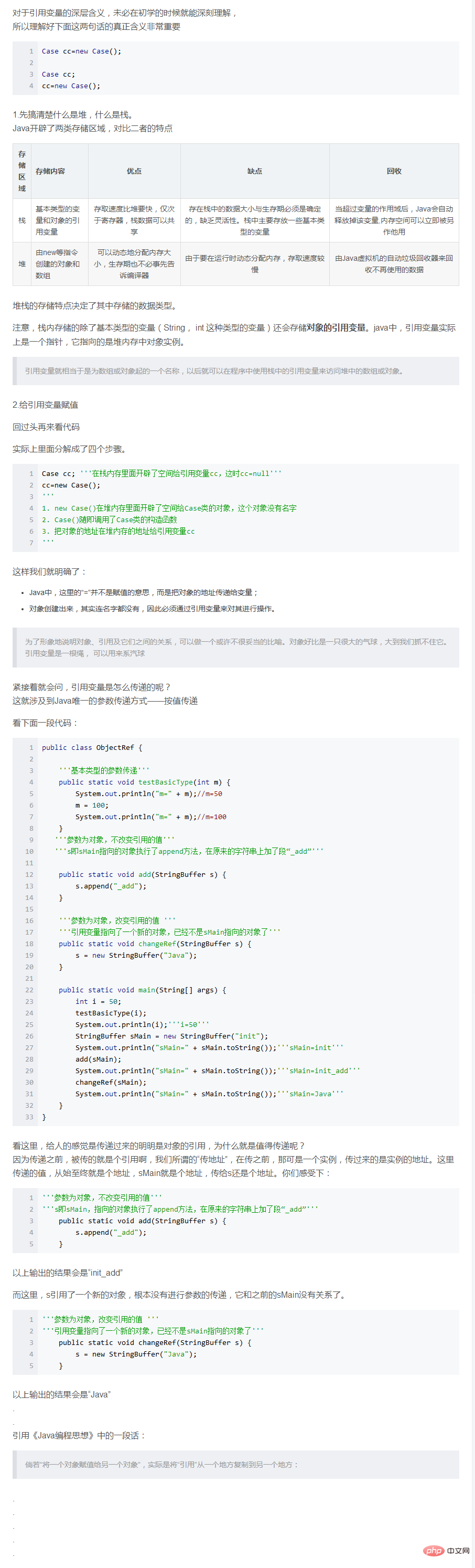# Java新手教程_java变量:

java变量就是可变的值

Java变量:

int:一般四个字节

``````int 数据类型是32位、有符号的以二进制补码表示的整数.

``````

### (转载的)为什么int类型的最大值是2^31-1 ？

int类型4个字节，1个字节等于8个比特，32位，最大值用二进制表示就是， 0111...(总共31个1)。为什么第一位是0？ 二进制里，最高位(第一位)表示符号

0表示正，1表示负。

2的n次方求和（n=30），由等比数列可知， 2^0 + 2^1 + 2^2...+2^n=2^(n+1)-1 = 2^31-1

1000(31个0)的补码还是本身。 所以最小值就是, 2^00 + 2^10 + 2^20...+ 2^311=2^31= -2^31

### 也是转载来的原文作者也不知道是谁了。